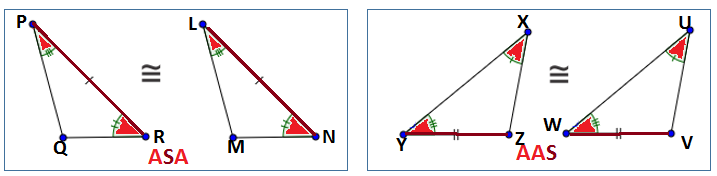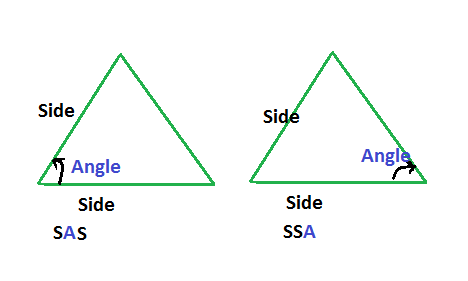D

#### Triangles

58 viewed last edited 6 years agoPuneet
0
What is the difference between ASA and AAS congruency criteria? Also SAS and SSA congruency criteria?Rounak Pathak
0
Asa is 2 angles and one side is congruent Sas is 2 sides and one angle is congruentPuneet
0Sriniketan
0
Consider triangle ABC. So, in ASA congruence criterion, angle A, side AB and angle B are equal to the corresponding parts of any other triangle. But in AAS congruence criterion, angle A, angle B and side BC are equal to the corresponding parts of the other triangle. However, if angle A and angle B are congruent, then angle C will also be congruent. Therefore, ASA congruence criterion and AAS congruence criterion are considerably same. Now, consider the same triangle ABC SO, in SAS congruence criterion, side AB, side BC and angle B are equal to the corresponding parts of the other triangle. SSA congruence criterion does not exist.Puneet
0
Thank youKrishna
0Congruent triangles: If two triangles are congruent, all three corresponding sides are congruent and all three corresponding angles are congruent. AAS and ASA are two theorems are Shortcut to know the two triangles are congruent or not. Angle-Side-Angle (ASA): Two pairs of corresponding angles and the "side between them" are known to be congruent, the triangles are congruent. This is known as angle-side-angle (ASA). Angle-Angle-Side (AAS): Two pairs of angles and the non-included side (the side not in between the angles) are known to be congruent, the triangles are congruent. this is known as angle-angle-side (AAS). Difference: See the figure in ASA theorem the PR, LN sides lie in between the angles. In AAS theorem the side TZ, WV sides are not between the angles. we can also take XZ, UV sides to prove AAS.Krishna
-1Difference: In SAS theorem the angle lies in between the sides. In SSA theorem the angle is not between the sides.Vivekanand Vellanki
0
There is no SSA congruence rule.Vivekanand Vellanki
0
There is no SSA congruence rule. See the discussion in https://www.qalaxia.com/#/viewDiscussion?messageId=59a51dfb7f173b981abee4a7 ASA congruence rule states that 2 angles and the included side are equal. Lets understand ASA congruence rule better. If 2 angles in 2 triangles are same, then all the 3 angles in the 2 triangles are the same. If the 3 angles are same, you can draw infinitely many such triangles. Draw one triangle ABC with the given angles. Extend the sides AB and AC. Now, draw a line DE parallel to BC - this intersects AB at D and AC at E. Clearly, although ABC and ADE have all 3 angles same, they are not congruent. To make the two triangles congruent, one corresponding side has to be equal in both triangles. If two triangles ABC and DEF have equal angles; and AB = DE; then the two triangles are congruent if: angle A = angle D angle B = angle E One can claim this is ASA congruence rule. Another person can claim this is by AAS congruence rule because: angle C = angle F, and angle A = angle D, and AB = DE If you find that two triangles have 2 angles same, look for corresponding sides to be equal to establish congruence. As an example, if angle A = angle D, and angle B = angle E, and AB = EF these 2 triangles are not congruent by AAS since AB and EF are not corresponding sides.